# 7 Receptor impact model estimation (stage 6)

Page 7 of 14

This stage constructs the receptor impact models given the specified model structure and the elicited responses contributed by experts, conditional on the hydrological scenarios presented at the receptor impact modelling workshop. The receptor impact models constructed for each landscape class within a bioregion or subregion are reported along with the relevant contextualisation in terms of landscape class definitions and qualitative modelling results in the corresponding product 2.7 (receptor impact modelling) (Figure 3). The method used for the receptor impact model construction is detailed below.

## 7.1 Prior distribution of beta

Conditional on a design point, the elicited subjective probability distribution is Gaussian,(34)

The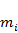andare assumed conditionally independent from other elicitations given the design matrix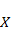. Letanddenote a diagonal covariance matrix. The conditional distribution ofis proportional to:(35)

The distribution for the unknownconditional on,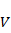andis then proportional to a multivariate normal with mean and covariance given by:(36)

## 7.2 Model structure uncertainty

The full design matrix allows for a quadratic surface response function between the hydrological response function and the receptor impact variables. This includes linear terms, pairwise interactions between the linear terms and quadratic terms for all hydrological response variables. If the hydrological response variables vary in the reference period, then an interaction between the quadratic surface response function and the future period is also included. The full design model structures the elicitation scenario so that this complex model can be elucidated.

However, the full richness of the model may be excessive for simple relationships between hydrological response variables and receptor impact variables. Simpler models are therefore considered. Optional model terms include: interactions between hydrological response variables and the future period, the pairwise interactions between different hydrological response variables and the quadratic terms. The alternative models are ranked using a Bayesian information criterion (BIC) (proportional to the Schwarz criterion; Schwarz, 1978) metric:(37)

whereis the dimension of the vector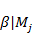and. The model with the lowest BIC value is selected as the best model.

Note that several terms are required to be retained within all the candidate models. These include: the intercept, the future-period factor, the short-term factor, the influence of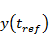and at least the linear terms of all hydrological response variables to be considered in the receptor impact modelling elicitation workshop. The inclusion of this minimal subset ensures that the covariates which provide the structure for the elicitation scenarios are also represented in the estimation and prediction steps of the receptor impact modelling.

Last updated:
18 October 2018